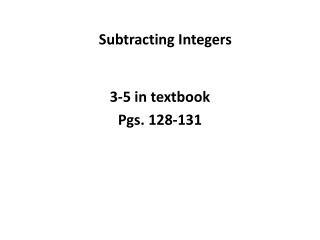DownloadDownload PresentationSubtracting Integers

# Subtracting Integers

Télécharger la présentation## Subtracting Integers

- - - - - - - - - - - - - - - - - - - - - - - - - - - E N D - - - - - - - - - - - - - - - - - - - - - - - - - - -
##### Presentation Transcript

1. Subtracting Integers 3-5 in textbook Pgs. 128-131

2. Review Adding Integers IF SAME SIGN: Add the numbers: Positive + Positive = Positive Negative + Negative = Negative Example: 4 + 3 = 7 OR (-3) + (-10) = -13

3. IF DIFFERENT SIGN: Subtract the numbers & sum has sign of biggest # Example: (-5) + 7 Subtract numbers: 7 – 5 = 2 7 is biggest # So sum is positive Answer = -2

4. Subtracting Integers Rule: Change the subtraction sign to addition. Change the sign of the 2nd number. Then ADD. Example: 8 - 13 8 + 13 1) Change subtraction sign to addition 8 + (-13) 2) Change the sign of the 2nd number 3) ADD *Adding integers with different signs *Subtract #’s 13 – 8 = 5 Take sign of biggest # Answer: -5

5. Example: -10 - 7 -10 + 7 1) Change the subtraction sign to addition 2) Change the sign of the 2nd number -10 + (-7) 3) Add Adding integers with SAME sign (negative + negative) 10 + 7 = 17 Sum is negative Answer = -17

6. Example: 1 – (-7) 1 + (-7) 1) Change subtraction sign to addition 2) Change sign of 2nd number 1 + 7 3) ADD. Adding integers with SAME sign (positive + positive = positive) 1 + 7 = 8 Answer = 8